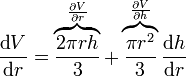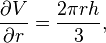Skip navigation

# Total derivatives vs Partial derivatives

So what’s the diff? Ha ha. Get it?

The total derivative is what you’re introduced to in elementary calculus.

Its the one where you have an equation like:

P(x) = 2*x2 + x

and then you take the (total) derivative with respect to x:

P'(x) = 4*x + 1

Intuitively a derivative is how fast something changes, as you change another thing.

So P'(x) describes the rate of change (how fast P(x) changes) as you change the x value.

So when x=2, P'(x)=9, which means P(x) is increasing fairly rapidly at x=2.

Now, take this formula for the Volume Of a Cone (imagine: the amount of water a dunce cap can hold or something).Here, there are two independent variables: the radius r and the height h.

Uh oh. That means there are two ways to change the volume of a cone!! One is by increasing its height (holding the width constant, so the dunce cap becomes taller) and the other is by increasing its width (holding the height constant, so making the cap fit a fatter dunce’s head).

Of course, we could ALSO make it so the HEIGHT of the hat DEPENDS ON the WIDTH of the hat. And THAT’S what the total derivative is “afraid of”. That’s why there are two terms in the total derivative, as we’ll see next:

Let’s take the total derivative of V with respect to r:Hey!! Why do we have that dh/dr term.

THAT dh/dr term is there JUST IN CASE there is a dependence of height (h) on radius (r). If we want the hat to always have the same cone-ish shape as we increase the width of the hat, (like we don’t want it to become a fat flat cone), then we have to make the HEIGHT of the hat DEPENDENT on the RADIUS.

Say we want the height of the cone to always be 3x the radius. Then we’d say:

h = 3r

Then

```dh
-- = 3
dr
```

If we DON’T care to maintain the same aspect ratio of the cone as we widen the hat, then we could say the height of the hat DOESN’T have a dependence on the width of the hat, then

```dh
-- = 0
dr
```

So in that case the total derivative of the volume of the hat with respect to radius reduces to

```2 PI r h
-------
3
```

(The PI r2/3 term vanished because dh/dr was 0).

So the TOTAL DERIVATIVE INCLUDES ACCOUNTING FOR THE CASE WHERE THERE IS DEPENDENCE OF ONE INDEPENDENT VARIABLE ON ANOTHER.

Partial derivatives don’t do that.

When you take the partial derivatives of volume with respect to radius, you get:So you can see that we assumed h is a CONSTANT as we take the derivative in r. This means we ignore any possible dependence h might have on r with the partial derivative.

I hate the blue ring in windows 7 pen input. Using a wacom tablet, I can

Kill WispTis.exe

Restart wacom driver

Voila, no blue ring on right click.

Inking doesn’t work anymore in this mode however.

# visual studio keyboard shortcut to collapse a region

To collapse the region that the cursor is currently in in Visual Studio 2010 just type CTRL+M, CTRL+S,

Edit.CollapseCurrentRegion

Collapses the region that contains the cursor to show just the top line of the region, followed by an ellipsis. Regions are indicated by triangles on the left edge of the document window